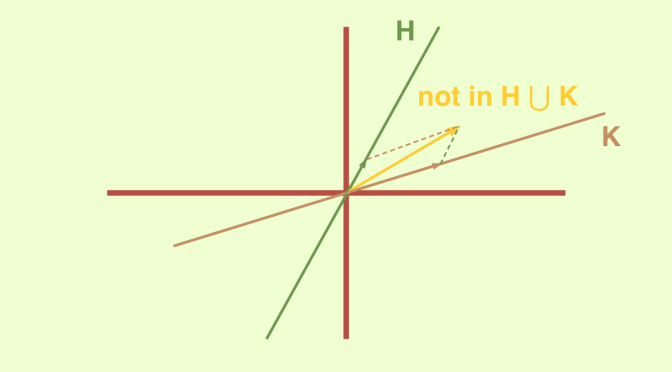# A vector space written as a finite union of proper subspaces

We raise here the following question: “can a vector space $$E$$ be written as a finite union of proper subspaces”?

Let’s consider the simplest case, i.e. writing $$E= V_1 \cup V_2$$ as a union of two proper subspaces. By hypothesis, one can find two non-zero vectors $$v_1,v_2$$ belonging respectively to $$V_1 \setminus V_2$$ and $$V_2 \setminus V_1$$. The relation $$v_1+v_2 \in V_1$$ leads to the contradiction $$v_2 = (v_1+v_2)-v_1 \in V_1$$ while supposing $$v_1+v_2 \in V_2$$ leads to the contradiction $$v_1 = (v_1+v_2)-v_2 \in V_2$$. Therefore, a vector space can never be written as a union of two proper subspaces.

We now analyze if a vector space can be written as a union of $$n \ge 3$$ proper subspaces. We’ll see that it is impossible when $$E$$ is a vector space over an infinite field. But we’ll describe a counterexample of a vector space over the finite field $$\mathbb{Z}_2$$ written as a union of three proper subspaces.

## The case of a vector space over an infinite field

We consider a vector space $$E$$ over an infinite field, for instance $$\mathbb{R}$$ or $$\mathbb{C}$$. Suppose that $$\displaystyle E= \bigcup_{k=1}^n V_k$$ is the union of $$n \ge 2$$ proper subspaces and that $$n$$ is minimal, i.e. that $$E$$ cannot be written as a union of less than $$n$$ subspaces where the subspaces are chosen in $$\{V_1, \dots,V_n\}$$. Thus in each $$V_k$$, there is a vector $$v_k$$ not in the union of the other $$V_{k^\prime}$$. In $$S=\{t v_1 + (1-t) v_2; 0 < t < 1 \}$$ there are infinitely many vectors and so two different ones among them must belong to some subspace, say $$V_{k^\prime}$$. But then $$S \subset V_{k^\prime}$$, hence the contradiction $$v_1,v_2 \in V_{k^\prime}$$.

## The case of a vector space over the finite field $$\mathbb{Z}_2$$

We now take for $$E$$ the “plane” $$\mathbb{Z}_2^2$$ and consider the three “lines” $$V_1=\mathbb{Z}_2 \cdot e_1$$, $$V_2=\mathbb{Z}_2 \cdot e_2$$ and $$V_3=\mathbb{Z}_2 \cdot (e_1+e_2)$$ where $$(e_1,e_2)$$ is the canonical basis.
$$E$$ is equal to the set of four vectors $$\{(0,0),(1,0),(0,1),(1,1)\}$$ and it is easy to verify that $$E=V_1 \cup V_2 \cup V_3$$.